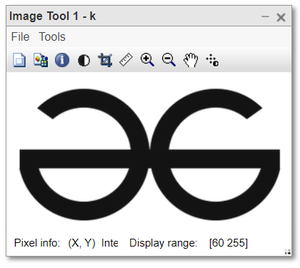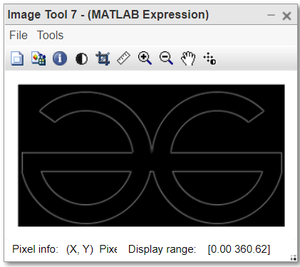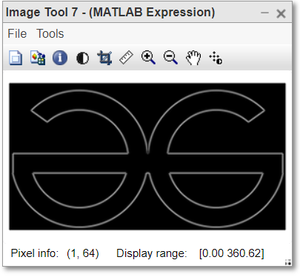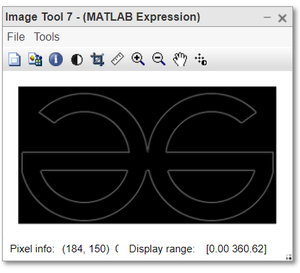# Edge detection using first derivative operator in MATLAB

• Last Updated : 16 Oct, 2021

An edge in an image is a significant local change in image intensity, usually associated with a discontinuity in image intensity or the first derivative of image intensity. The discontinuities in the intensity of the image can be stepped discontinuities, in which the intensity of the image changes from one value on one side of the discontinuity to a different value on the opposite side, or a line discontinuity. , in which the intensity The image changes in value abruptly but then returns to the original value for a short distance. However, step and line edges are rare in actual images. Due to the low-frequency components or smoothing introduced by most detection devices, there are rarely any sharp discontinuities in the actual signals. The edges of the steps become the edges of the ramps, and the edges of the lines become the edges of the roof, where changes in intensity are not instantaneous but occur over a finite distance.

The discontinuity in the image brightness is called an edge.

Edge detection is the technique used to identify the regions in the image where the brightness of the image changes sharply. This sharp change in the intensity value is observed at the local minima or local maxima in the image histogram, using the first-order derivative.

Now we detect edge using the first derivative operator with different coordinates. To detect an edge using the forward operator we use given below steps:

Steps:

• Convert into grayscale if it is colored.
• Convert into the double format.
• Define the mask or filter.
• Detect the edges along X-axis.
• Detect the edges along Y-axis.
• Combine the edges detected along X and Y axes.
• Display all the images.

Syntax:

var_name = imread(” name of image . extension “);

var_name = rgb2gray ( old_image_var);

var_name = double(image);

Final_image = sqrt ( Kx.^2 + Ky.^2);

imtool(image, []);

imtool(abs(image), []);

Imtool() is the inbuilt function in Matlab. It is used to display the image. It takes 2 parameters; the first is the image variable and the second is the range of intensity values. We provide an empty list as the second argument which means the complete range of intensity has to be used while displaying the image.

Example 1:

## Matlab

 `% MATLAB code for Forward  ` `% operator edge detection ` `k=imread(``"logo.png"``); ` `k=rgb2gray(k); ` `k1=double(k); ` `forward_msk=[1 -1 0]; ` `kx=conv2(k1, forward_msk, ``'same'``); ` `ky=conv2(k1, forward_msk``', '``same'); ` `ked=sqrt(kx.^2 + ky.^2); ` ` `  `%display the images. ` `imtool(k,[]); `   `%display the edge detection along x-axis. ` `imtool(kx,[]); ` `imtool(abs(kx), []); ` ` `  `%display the edge detection along y-axis. ` `imtool(ky,[]); ` `imtool(abs(ky),[]); ` ` `  `%display the full edge detection. ` `imtool(ked,[]); ` `imtool(abs(ked),[]); `

Output:gray image.Edge detected image

Example 2:

## Matlab

 `% MATLAB code for Central ` `% operator edge detection ` ` ``k=imread(``"logo.png"``); ` ` ``k=rgb2gray(k); ` ` ``k1=double(k); ` ` ``central_msk=[1/2 0 -1/2]; ` ` ``kx=conv2(k1, central_msk, ``'same'``); ` ` ``ky=conv2(k1, central_msk``', '``same'); ` ` ``ked=sqrt(kx.^2 + ky.^2); ` `  `  ` ``%display the images. ` ` ``imtool(k,[]); ` `  `  ` ``%display the edge detection along x-axis. ` ` ``imtool(kx,[]); ` ` ``imtool(abs(kx), []); ` `  `  ` ``%display the edge detection along y-axis. ` ` ``imtool(ky,[]); ` ` ``imtool(abs(ky),[]); ``%binarised form ` `  `  ` ``%display the full edge detection. ` ` ``imtool(abs(ked),[]); `

Output:

Here, we can observe that the edge is more clearly visible.Gray imageEdge detected image

Example 3:

## Matlab

 `% MATLAB code for Backward ` `% operator edge detection ` `k=imread(``"logo.png"``); ` `k=rgb2gray(k); ` `k1=double(k); ` `backward_msk=[0 1 -1]; ` `kx=conv2(k1, backward_msk, ``'same'``); ` `ky=conv2(k1, backward_msk``', '``same'); ` `ked=sqrt(kx.^2 + ky.^2); `   `%display the images. ` `imtool(k,[]); `   `%display the edge detection along x-axis. ` `imtool(kx,[]); ` `imtool(abs(kx), []); `   `%display the edge detection along y-axis. ` `imtool(ky,[]); ` `imtool(abs(ky),[]); `   `%display the full edge detection. ` `imtool(ked,[]); ` `imtool(abs(ked),[]);`

Output:Original imageEdge detected image

My Personal Notes arrow_drop_up
Recommended Articles
Page :